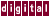## 4.5.19 /unroll, /ur, (unroll=4), /unroll2, /ur2, (/unroll2=160), /unroll3, /ur3, (/unroll3=1)

The `/unroll, /unroll2,` and `/unroll3` qualifiers modify how KAP unrolls inner loops. More work per iteration with fewer iterations gives less overhead. The `/scalaropt=2` qualifier level is required to enable inner loop unrolling. Each iteration reaches the value given by the `/unroll2` qualifier.

Note
If you use `kapc` with the DEC C compiler optimization qualifier set to `O5` , you should turn off KAP's loop unrolling by setting `/unroll=1`

Outer loop unrolling is performed as part of memory management and is not controlled by these qualifiers.

The syntax for `/unroll` and `/unroll2` is as follows:

```Long forms:   /unroll=<#IT> or /unroll2=<WEIGHT>

Short forms:  /ur=<#IT> or /ur2=<WEIGHT>
```

Where:

`<#IT>` is the maximum number of iterations to unroll.
`=0` uses default values to unroll.
`=1` means no unrolling.
`<WEIGHT>` is the maximum weight, that is, estimate of work, in an unrolled loop. `<WEIGHT>` is estimated by counting operands and operators in a loop.

There are two ways to control loop unrolling. The first is to set the maximum number of iterations that can be unrolled; the second is to set the maximum amount of work to be done in an unrolled iteration. KAP will unroll as many iterations as possible while keeping within both these limits, up to a maximum of 100 iterations. NO warning is given if you request more than 100 unrolled iterations.

The default (4,200) means that the maximum number of iterations to unroll is 4 and that the maximum amount of work is 200.

By increasing or decreasing the maximum iteration workload, you can control the amount of work that ends up in each loop iteration, as long as the number of unrolled iterations does not exceed the unroll limit. The workload is estimated by adding operations, including subscripts and assignments; scalars, not including the loop index; and `if` statements. Loops with function calls are weighted more heavily and are never unrolled. The following example demonstrates the workload limit. Assume that `/unroll=3` and `/unroll2=24` are the qualifier settings.

```for ( i=0; i<n; i++ ) {
a[i] = b[i]+c[i];
}
```

The amount of work in this loop is 5. By default, the loop would be unrolled three times, because that is the maximum allowed by the unroll limit, and the resulting weight (3*5) is less than the `unroll2` limit of 24.

If you set the `/unroll2` limit to 10, the loop would be unrolled twice because unrolling the original loop three times would produce a loop with workload of 15, which would exceed the ``` /unroll2``` limit. The result would be the following:

```for ( i = 0; i<=n - 2; i+=2 ) {
a[i] = b[i] + c[i];
a[i+1] = b[i+1] + c[i+1];
}
for ( ; i<n; i++ ) {
a[i] = b[i] + c[i];
}
```

The `unroll3=n` qualifie sets the lower limit for unrolling. If there are less than `n` units of work in the loop (same units as `/unroll2` ), the loop will not be unrolled. The amount of work in each loop iteration is shown in the loop table in the annotated listing. This qualifier value should be left at 1, the default.

Previous Page Next Page Contents Index
Command-Line Qualifiers